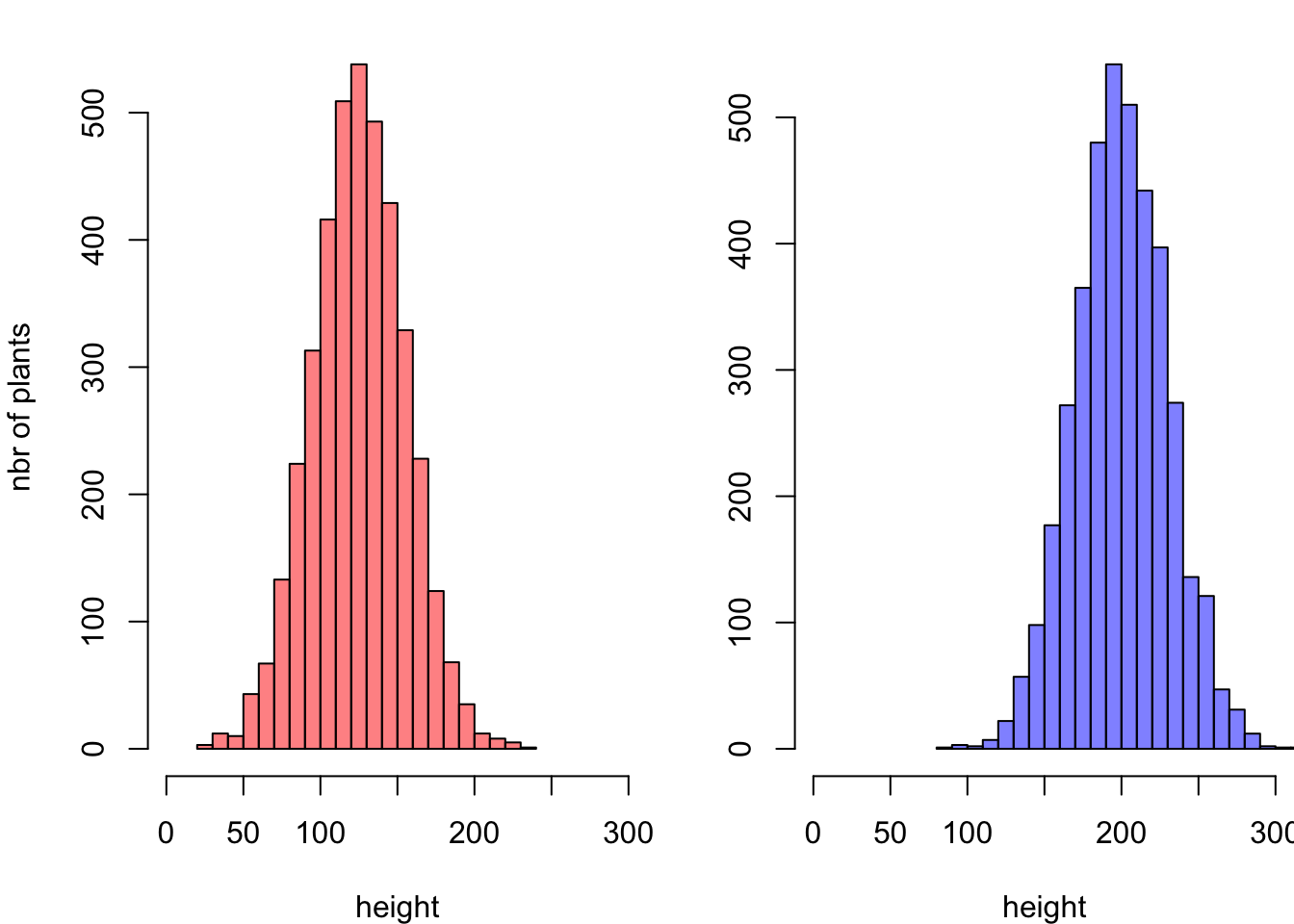# Two Histograms with melt colors

A histogram displays the distribution of a numeric variable. This posts explains how to plot 2 histograms on the same axis in Basic R, without any package.

Histogramms are commonly used in data analysis to observe distribution of variables. A common task in data visualization is to compare the distribution of 2 variables simultaneously.

Here is a tip to plot 2 histograms together (using the `add` function) with transparency (using the rgb function) to keep information when shapes overlap.``````#Create data
set.seed(1)
Ixos=rnorm(4000 , 120 , 30)

# First distribution
hist(Ixos, breaks=30, xlim=c(0,300), col=rgb(1,0,0,0.5), xlab="height",
ylab="nbr of plants", main="distribution of height of 2 durum wheat varieties" )

# Second with add=T to plot on top

rgb(0,0,1,0.5)), pt.cex=2, pch=15 )``````

Note: this is how the figure looks like if groups are drawn one beside each other:

``````par(
mfrow=c(1,2),
mar=c(4,4,1,0)
)

hist(Ixos, breaks=30 , xlim=c(0,300) , col=rgb(1,0,0,0.5) , xlab="height" , ylab="nbr of plants" , main="" )
hist(Primadur, breaks=30 , xlim=c(0,300) , col=rgb(0,0,1,0.5) , xlab="height" , ylab="" , main="")``````Related chart types

## Contact

This document is a work by Yan Holtz. Any feedback is highly encouraged. You can fill an issue on Github, drop me a message on Twitter, or send an email pasting yan.holtz.data with gmail.com.